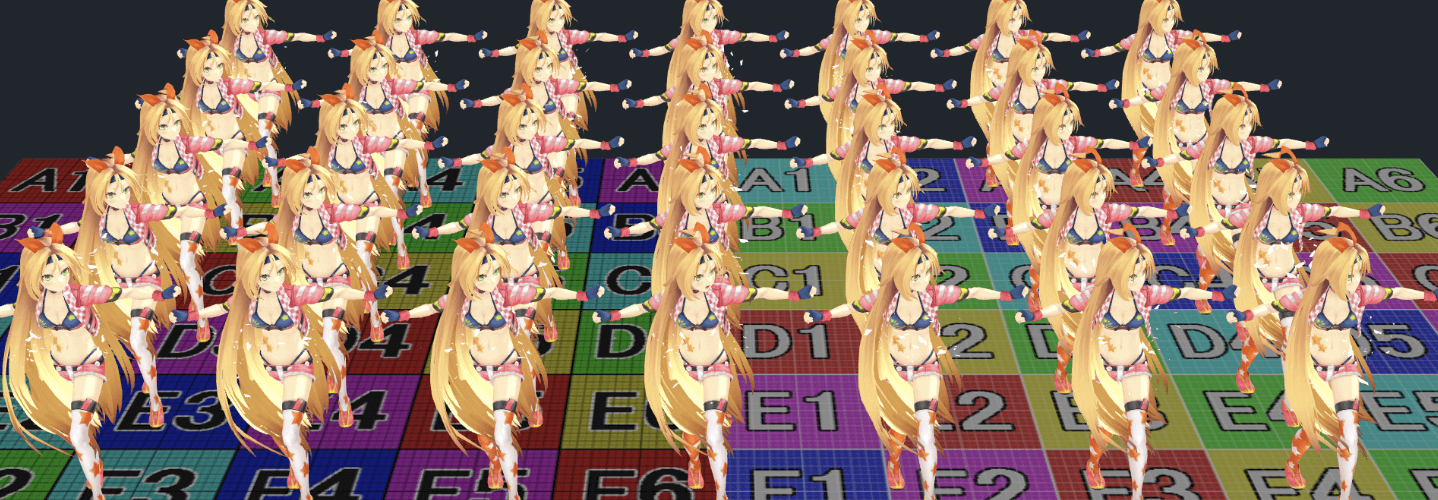# uSpringBone开源库测评

## 一、介绍

uSpringBone链接：https://lab.uwa4d.com/lab/5bf67e9d72745c25a836b5ca

## 二、项目解读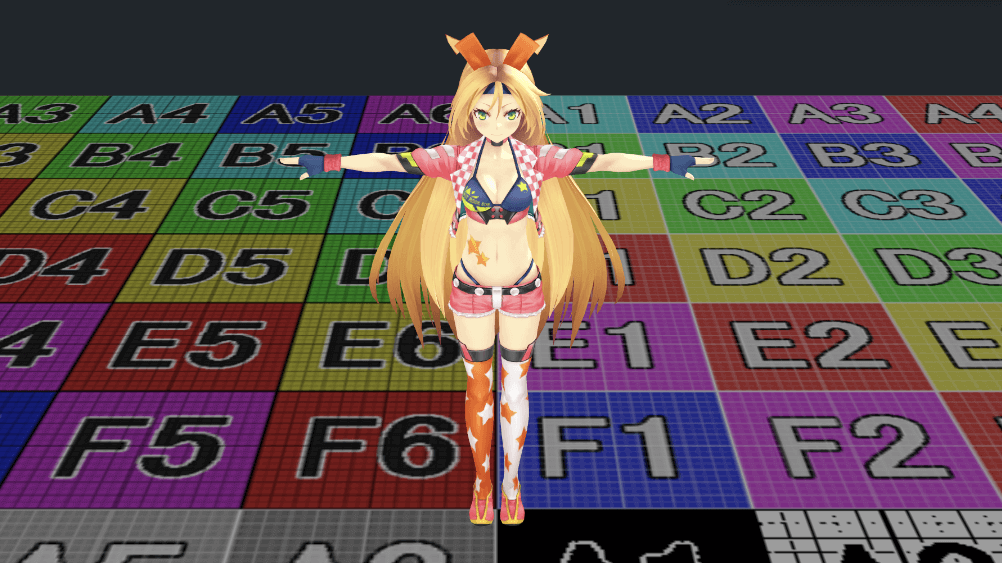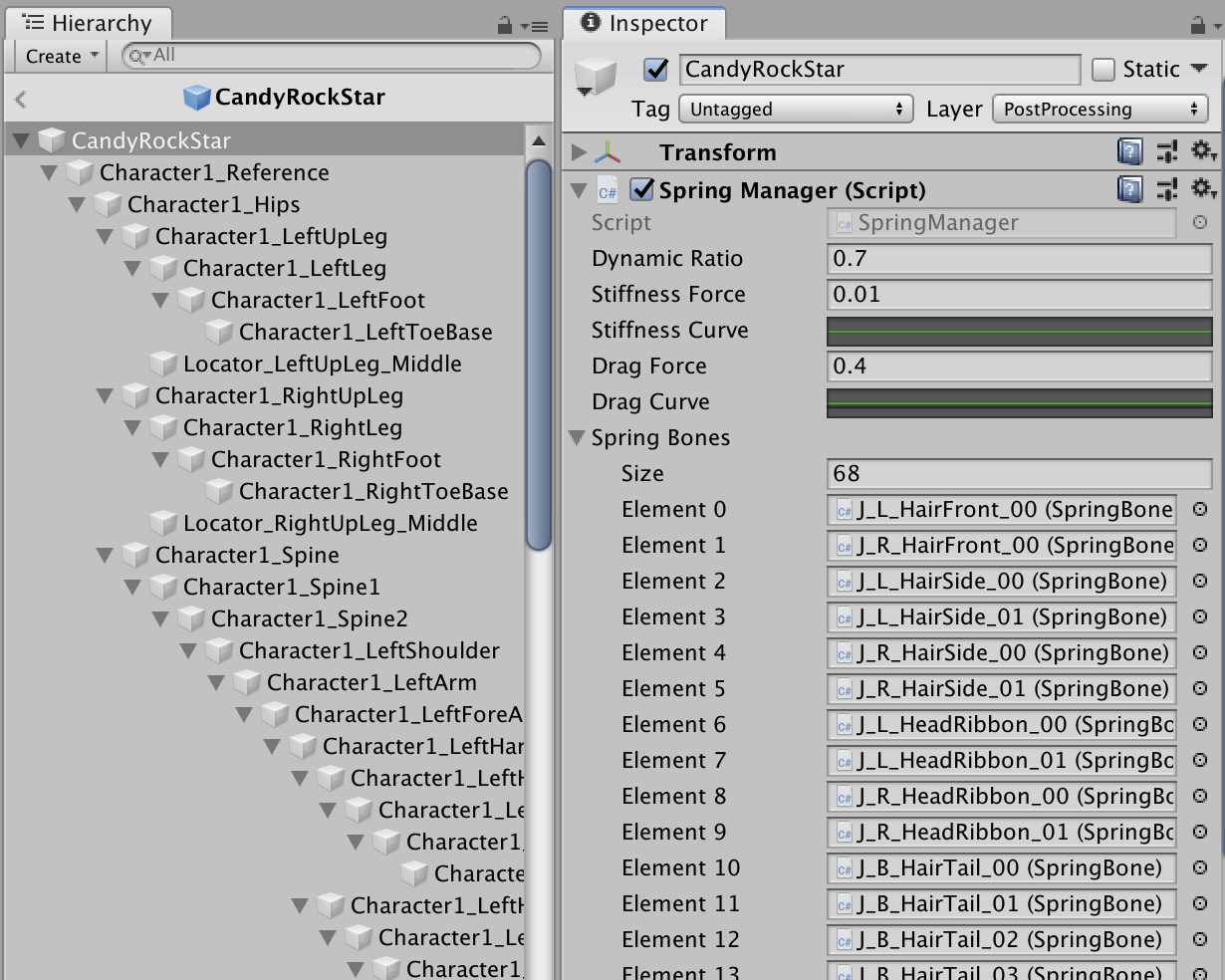• SphereColliderComponent：球形碰撞体组件，用于添加到需要计算碰撞的必要节点上。当然，由于要用Job System来加速，这必须是一个IComponentData类型的数据结构。
• SpringBoneComponent：弹簧骨骼组件，用于添加到需要应用弹簧效果的骨骼节点上，同样这也是一个IComponentData类型的数据。
• SpringBoneChain：弹簧骨骼管理组件，用于添加到一个所有SpringBone的父节点上。
• SpringBoneJobScheduler：SpringBone的运行进程管理脚本。

SpringBoneChain.cs中的Execute方法的核心代码如下：

``````// spring.
for (int i = 0; i<boneData.Length; ++i)
{
var bone = boneData[i];

// set root parent data.
...

// get local and grobal position.
...

// calculate force.
float3 force = mul(grobalRotation, (bone.boneAxis * bone.stiffnessForce)) / sqrDt;
force += (bone.previousEndpoint - bone.currentEndpoint) * bone.dragForce / sqrDt;
force += bone.springForce / sqrDt;

float3 temp = bone.currentEndpoint;
var dataTemp = boneData[i];

// calculate next endpoint position.
dataTemp.currentEndpoint = (dataTemp.currentEndpoint - dataTemp.previousEndpoint) + dataTemp.currentEndpoint + (force* sqrDt);
dataTemp.currentEndpoint = (normalize(dataTemp.currentEndpoint - grobalPosition) * dataTemp.springLength) + grobalPosition;

// collision.
for (int j = 0; j<selectedColliderList.Length; ++j)
{
var collider = colliderData[j];
var colliderPosition = position[selectedColliderList[j].entity].Value;
{
float3 normal = normalize(dataTemp.currentEndpoint - colliderPosition);
dataTemp.currentEndpoint = (normalize(dataTemp.currentEndpoint - grobalPosition) * dataTemp.springLength) + grobalPosition;
}
}

dataTemp.previousEndpoint = temp;

// calculate next rotation.
float3 from = mul(parentRotation, bone.boneAxis);
float3 to = dataTemp.currentEndpoint - grobalPosition;
float diff = length(from - to);
quaternion targetRotation = Quaternion.identity;
if(float.MinValue<diff && diff<float.MaxValue)
targetRotation = Quaternion.FromToRotation(from, to);

// set calculated data.
dataTemp.grobalPosition = parentPosition + mul(parentRotation, localPosition);
dataTemp.grobalRotation = Quaternion.Lerp(dataTemp.grobalRotation, mul(targetRotation, parentRotation), 1f); // TODO: lerp parameter
parentPosition = dataTemp.grobalPosition;
parentRotation = dataTemp.grobalRotation;

// update data.
...

// update entity.
...
}
``````

``````[ScriptExecutionOrder(-32000)]
public class SpringBoneJobScheduler : MonoBehaviour
{
...
}
``````

## 三、性能测评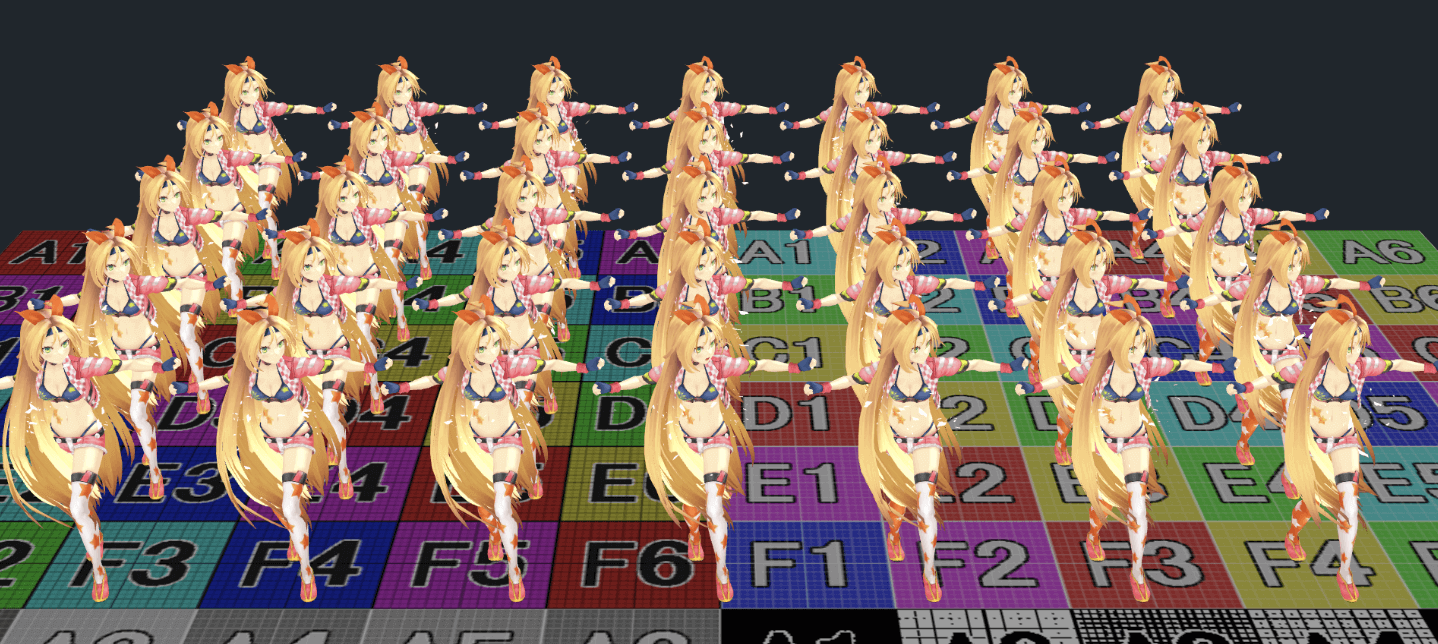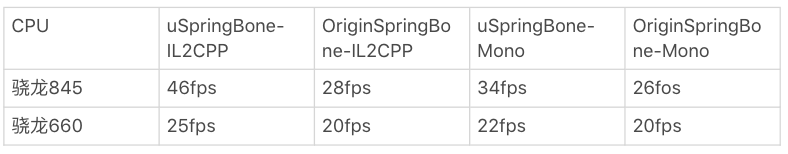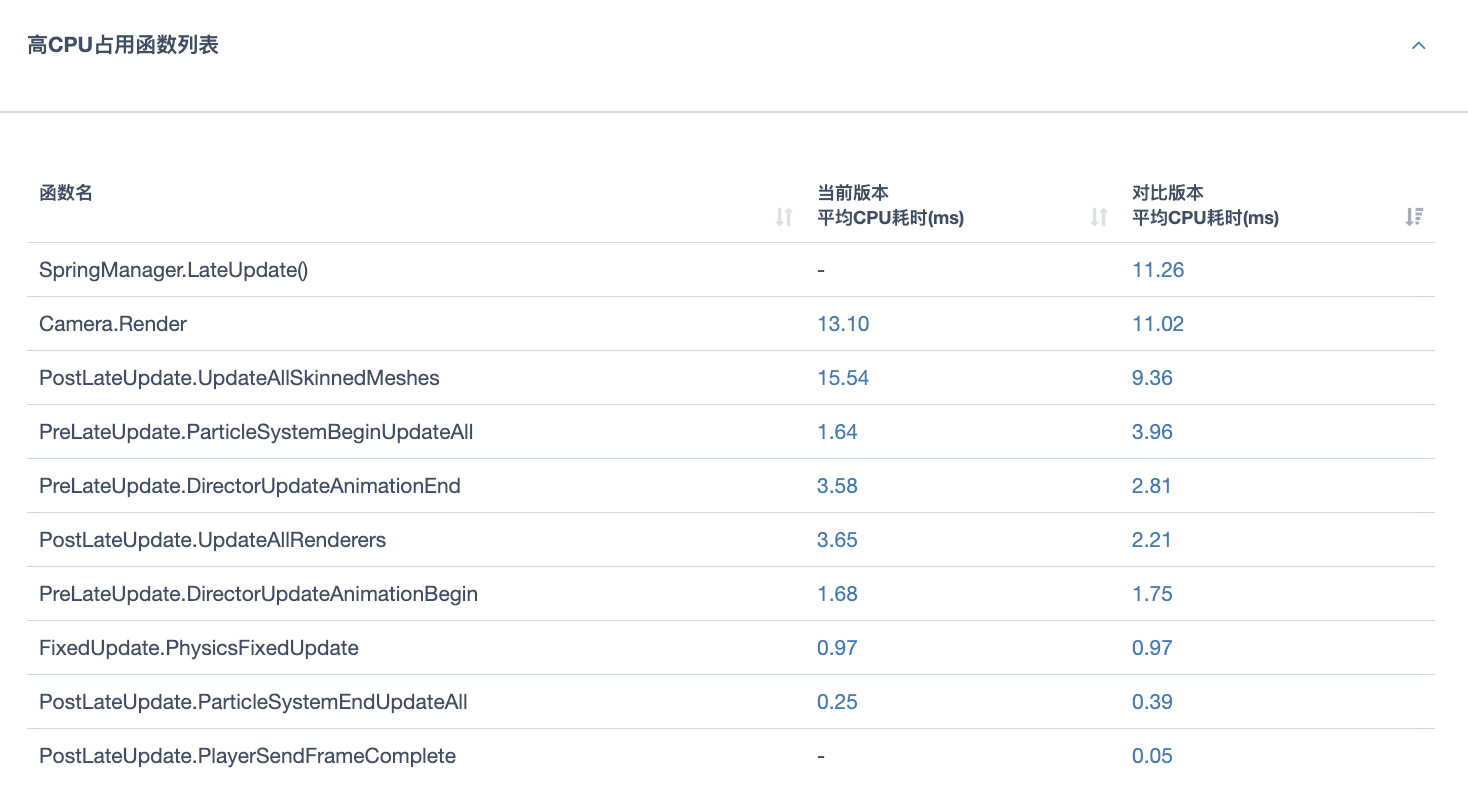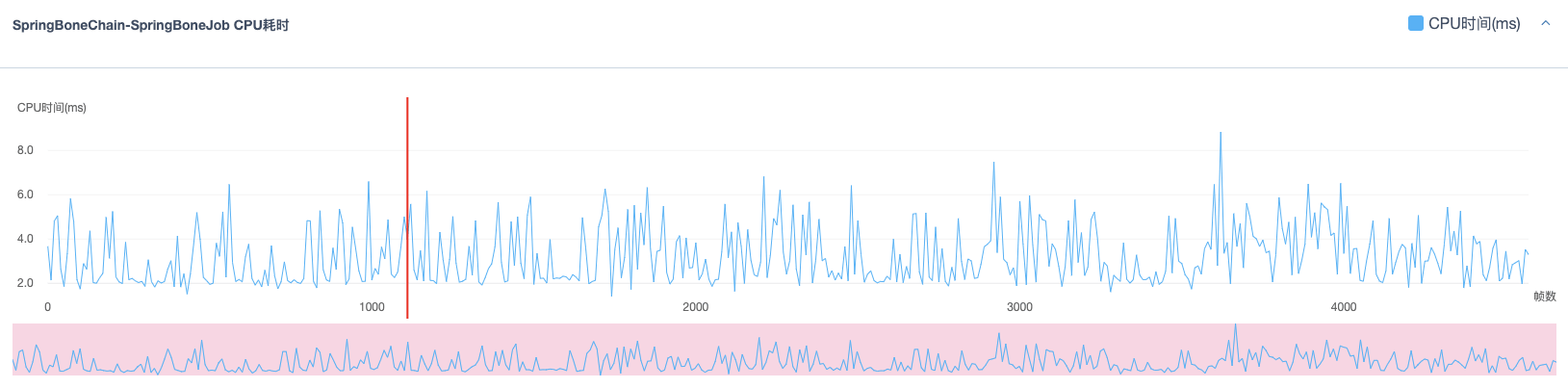## 四、总结

uSpringBone是一个应用Dots优化计算密集型逻辑耗时的实践，可以看到改造过程并不复杂，性能优化效果却很明显。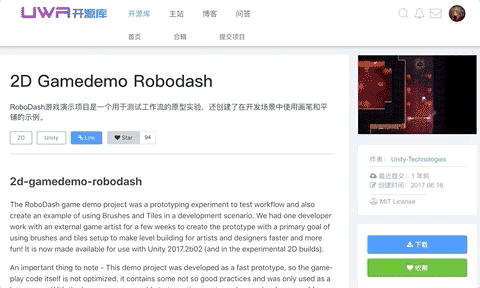【博物纳新】是UWA旨在为开发者推荐新颖、易用、有趣的开源项目，帮助大家在项目研发之余发现世界上的热门项目、前沿技术或者令人惊叹的视觉效果，并探索将其应用到自己项目的可行性。很多时候，我们并不知道自己想要什么，直到某一天我们遇到了它。